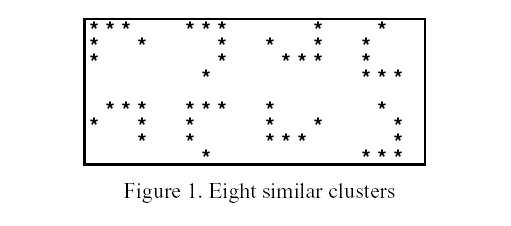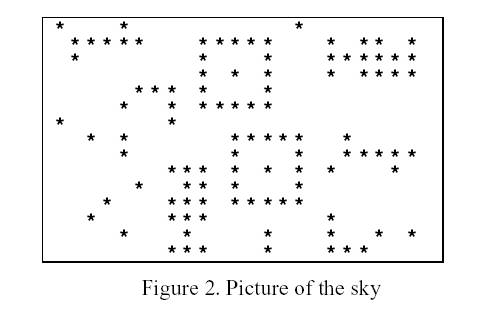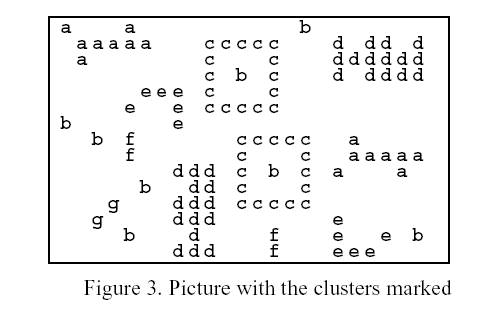# POJ1175:Starry Night(bfs)

http://poj.org/problem?id=1175

#include <iostream>
#include <stdio.h>
#include <string.h>
#include <stdlib.h>
#include <algorithm>
#include <queue>
#include <math.h>
#define MIN 1e-8
using namespace std;
int map;
char ff;
int n,m,tt,v;
struct node
{
int x,y;
};
int sum;
double dis;
struct node1
{
int x,y;
} s;
int jx[]= {1,1,1,0,0,-1,-1,-1};
int jy[]= {-1,0,1,-1,1,-1,0,1};
void bfs(int xx,int yy)
{
memset(v,0,sizeof(v));
queue<node>q;
struct node t,f;
int l=0;
s[l].x=xx;
s[l++].y=yy;
t.x=xx;
t.y=yy;
v[t.x][t.y]=1;
q.push(t);
while(!q.empty())
{
t=q.front();
q.pop();
for(int i=0; i<8; i++)
{
f.x=t.x+jx[i];
f.y=t.y+jy[i];
if(map[f.x][f.y]&&v[f.x][f.y]==0&&f.x>=0&&f.x<n&&f.y>=0&&f.y<m)
{
s[l].x=f.x;
s[l++].y=f.y;
v[f.x][f.y]=1;
q.push(f);
}
}
}
double count=0;
for(int i=0; i<l; i++)
{
for(int j=0; j<l; j++)
{
count=count+sqrt((s[i].x-s[j].x)*(s[i].x-s[j].x)*1.0+(s[i].y-s[j].y)*(s[i].y-s[j].y)*1.0);
}
}
int flag=0;
for(int i=0; i<tt; i++)
{
if(sum[i]==l&&fabs(dis[i]-count)<MIN)
{
ff[xx][yy]=i+'a';
flag=1;
}
}
if(flag==0)
{
ff[xx][yy]=tt+'a';
dis[tt]=count;
sum[tt]=l;
tt++;
}
}
int main()
{
char str;
while(scanf("%d%d",&m,&n)!=EOF)
{
tt=0;
for(int i=0; i<n; i++)
{
getchar();
for(int j=0; j<m; j++)
{
scanf("%c",&str);//不知道为什么不能直接输入map[i][j],基础知识太渣了
ff[i][j]=str;
map[i][j]=str-'0';
}
}
for(int i=0; i<n; i++)
{
for(int j=0; j<m; j++)
{
if(map[i][j])
bfs(i,j);
}
}
for(int i=0; i<n; i++)
{
for(int j=0; j<m; j++)
{
printf("%c",ff[i][j]);
}
printf("\n");
}
}
return 0;
}

Description

High up in the night sky, the shining stars appear in clusters of various shapes. A cluster is a non-empty group of neighbouring stars, adjacent in horizontal, vertical or diagonal direction. A cluster cannot be a part of a larger cluster.

Clusters may be similar. Two clusters are similar if they have the same shape and number of stars, irrespective of their orientation. In general, the number of possible orientations for a cluster is eight, as Figure 1 exemplifies.The night sky is represented by a sky map, which is a two-dimensional matrix of 0's and 1's. A cell contains the digit 1 if it has a star, and the digit 0 otherwise.

Given a sky map, mark all the clusters with lower case letters. Similar clusters must be marked with the same letter; non-similar clusters must be marked with different letters. You mark a cluster with a lower case letter by replacing every 1 in the cluster by that lower case letter. The order of allocation of letters depends on the first appearance of different clusters, from top to bottom and from left to right.

Input

Your program is to read from standard input. The first two lines contain, respectively, the width W and the height H of a sky map. The sky map is given in the following H lines, of W characters each.

0 <= W (width of the sky map) <= 100
0 <= H (height of the sky map) <= 100
0 <= Number of clusters <= 500
0 <= Number of non-similar clusters <= 26 (a..z)
1 <= Number of stars per cluster <= 160

Output

Your program is to write to standard output. The output contains the same map as the input, except that the clusters are marked as described above.

Sample Input

23
15
10001000000000010000000
01111100011111000101101
01000000010001000111111
00000000010101000101111
00000111010001000000000
00001001011111000000000
10000001000000000000000
00101000000111110010000
00001000000100010011111
00000001110101010100010
00000100110100010000000
00010001110111110000000
00100001110000000100000
00001000100001000100101
00000001110001000111000

Sample Output

a000a0000000000b0000000
0aaaaa000ccccc000d0dd0d
0a0000000c000c000dddddd
000000000c0b0c000d0dddd
00000eee0c000c000000000
0000e00e0ccccc000000000
b000000e000000000000000
00b0f000000ccccc00a0000
0000f000000c000c00aaaaa
0000000ddd0c0b0c0a000a0
00000b00dd0c000c0000000
000g000ddd0ccccc0000000
00g0000ddd0000000e00000
0000b000d0000f000e00e0b
0000000ddd000f000eee000

Hint

Just to make it clearer, notice that this sample input corresponds to the following picture of the sky.Notice that this sample output corresponds to the following picture.Source

posted @ 2014-10-09 19:11  人艰不拆_zmc  阅读(373)  评论(0编辑  收藏